### The Likelihood Ratio Test

The likelihood ratio test (LRT) compares the likelihoods of two models where parameter estimates are obtained in two parameter spaces, the space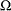and the restricted subspace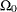. In the GLIMMIX procedure, the full model definesand the test-specification in the COVTEST statement determines the null parameter space. The likelihood ratio procedure consists of the following steps (see, for example, Bickel and Doksum 1977, p. 210):

1. Find the estimate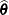of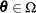. Compute the likelihood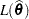.

2. Find the estimate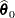of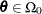. Compute the likelihood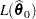.

3. Form the likelihood ratio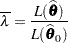4. Find a function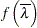that has a known distribution.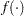serves as the test statistic for the likelihood ratio test.

Please note the following regarding the implementation of these steps in the COVTEST statement of the GLIMMIX procedure.

• The functionin step 4 is always taken to be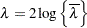which is twice the difference between the log likelihoods for the full model and the model under the COVTEST restriction.

• For METHOD=RSPL and METHOD=RMPL, the test statistic is based on the restricted likelihood.

• For GLMMs involving pseudo-data, the test statistics are based on the pseudo-likelihood or the restricted pseudo-likelihood and are based on the final pseudo-data.

• The parameter spacefor the full model is typically not an unrestricted space. The GLIMMIX procedure imposes boundary constraints for variance components and scale parameters, for example. The specification of the subspacemust be consistent with these full-model constraints; otherwise the test statistic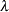does not have the needed distribution. You can remove the boundary restrictions with the NOBOUND option in the PROC GLIMMIX statement or the NOBOUND option in the PARMS statement.